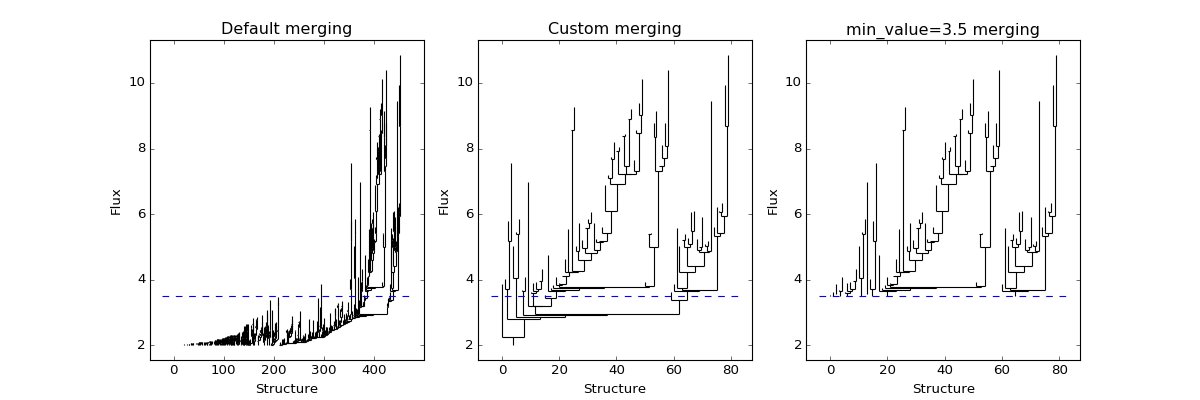## Specifying a custom structure merging strategy¶

By default, the decision about whether a leaf remains independent (i.e., whether it remains a leaf or its pixels get incorporated into another branch) when merged is made based on the `min_delta` and `min_npix` parameters, but in some cases, you may want to use more specialized criteria. For example, you may want only leaves overlapping with a certain position, or you may want leaves with a certain spatial or velocity extent, or a minimum peak value, to be considered independent structures.

In order to accomodate this, the `compute()` method can optionally take an `is_independent` argument which should be a function with the following call signature:

```def is_independent(structure, index=None, value=None):
...
```

where `structure` is the `Structure` object that is being considered, and `index` and `value` are the pixel index and value of the pixel that is linking the structure to the rest of the tree. These last two values are only set when calling the `is_independent` function during the tree computation, but the `is_independent` function is also used at the end of the computation to prune leaves that are not attached to the tree, and in this case `index` and `value` are not set.

The following example compares the dendrogram obtained with and without a custom `is_independent` function:

```import matplotlib.pyplot as plt
from astropy.io import fits
from astrodendro import Dendrogram

image = fits.getdata('PerA_Extn2MASS_F_Gal.fits')

fig = plt.figure(figsize=(15,5))

# Default merging strategy

d1 = Dendrogram.compute(image, min_value=2.0)
p1 = d1.plotter()

p1.plot_tree(ax1, color='black')
ax1.hlines(3.5, *ax1.get_xlim(), color='b', linestyle='--')
ax1.set_xlabel("Structure")
ax1.set_ylabel("Flux")
ax1.set_title("Default merging")

# Require minimum peak value
# this is equivalent to
# custom_independent = astrodendro.pruning.min_peak(3.5)
def custom_independent(structure, index=None, value=None):
peak_index, peak_value = structure.get_peak()
return peak_value > 3.5

d2 = Dendrogram.compute(image, min_value=2.0,
is_independent=custom_independent)
p2 = d2.plotter()

p2.plot_tree(ax2, color='black')
ax2.hlines(3.5, *ax2.get_xlim(), color='b', linestyle='--')
ax2.set_xlabel("Structure")
ax2.set_ylabel("Flux")
ax2.set_title("Custom merging")

# For comparison, this is what changing the min_value does:
d3 = Dendrogram.compute(image, min_value=3.5)
p3 = d3.plotter()

p3.plot_tree(ax3, color='black')
ax3.hlines(3.5, *ax3.get_xlim(), color='b', linestyle='--')
ax3.set_xlabel("Structure")
ax3.set_ylabel("Flux")
ax3.set_title("min_value=3.5 merging")
ax3.set_ylim(*ax2.get_ylim())
```Several pre-implemented functions suitable for use as `is_independent` tests are provided in `astrodendro.pruning`. In addition, the `astrodendro.pruning.all_true()` function can be used to combine several criteria. For example, the following code builds a dendrogram where each leaf contains a pixel whose value >=20, and whose pixels sum to >= 100:

```from astrodendro.pruning import all_true, min_peak, min_sum

custom_independent = all_true((min_peak(20), min_sum(100)))
Dendrogram.compute(image, is_independent=custom_independent)
```

You can specify custom neighbour logic by providing a `neighbours` function to `Dendrogram.compute()`. For example, the `periodic_neighbours()` utility will wrap neighbours across array edges. To correctly compute dendrograms for all-sky Cartesian maps:
```periodic_axis = 1  # data wraps along longitude axis